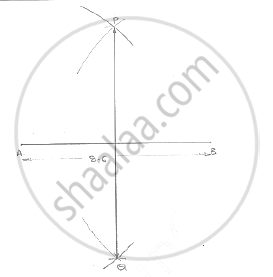# Draw a Line Segment of Length 8.6 Cm. Bisect It and Measure the Length of Each Part. - Mathematics

Draw a line segment of length 8.6 cm. Bisect it and measure the length of each part.

#### SolutionSteps of construction:
1. Draw a line segment AB of 86cm
2. With center A and radius more than n 1/2 AB,draw arcs, one on each side of AB
3. With center B and same radius, draw arcs cutting the previous arcs at P and Q respectively
4. Join PQ
∴ AC =  BC = 4.3cm

Concept: Introduction of Constructions
Is there an error in this question or solution?

#### APPEARS IN

RD Sharma Mathematics for Class 9
Chapter 16 Constructions
Exercise 16.1 | Q 1 | Page 3
Share